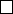1Groups and homomorphisms

IA Groups1.3 Cyclic groups
The simplest class of groups is cyclic groups. A cyclic group is a group of the
form
{e, a, a
2
, a
2
, · · · , a
n1
}
, where
a
n
=
e
. For example, if we consider the
group of all rotations of a triangle, and write
r
= rotation by 120
, the elements
will be {e, r, r
2
} with r
3
= e.
Officially, we define a cyclic group as follows:
Definition (Cyclic group C
n
). A group G is cyclic if
(a)(b)(n Z) b = a
n
,
i.e. every element is some power of a. Such an a is called a generator of G.
We write C
n
for the cyclic group of order n.
Example.
(i) Z is cyclic with generator 1 or 1. It is the infinite cyclic group.
(ii) ({+1, 1}, ×) is cyclic with generator 1.
(iii) (Z
n
, +) is cyclic with all numbers coprime with n as generators.
Notation.
Given a group
G
and
a G
, we write
hai
for the cyclic group
generated by
a
, i.e. the subgroup of all powers of
a
. It is the smallest subgroup
containing a.
Definition
(Order of element)
.
The order of an element
a
is the smallest integer
n
such that
a
n
=
e
. If
n
doesn’t exist,
a
has infinite order. Write
ord
(
a
) for the
order of a.
We have given two different meanings to the word “order”. One is the order
of a group and the other is the order of an element. Since mathematicians
are usually (but not always) sensible, the name wouldn’t be used twice if they
weren’t related. In fact, we have
Lemma. For a in g, ord(a) = |hai|.
Proof.
If
ord
(
a
) =
,
a
n
6
=
a
m
for all
n 6
=
m
. Otherwise
a
mn
=
e
. Thus
|hai| = = ord(a).
Otherwise, suppose
ord
(
a
) =
k
. Thus
a
k
=
e
. We now claim that
hai
=
{e, a
1
, a
2
, · · · a
k1
}
. Note that
hai
does not contain higher powers of
a
as
a
k
=
e
and higher powers will loop back to existing elements. There are also no repeating
elements in the list provided since a
m
= a
n
a
mn
= e. So done.
It is trivial to show that
Proposition. Cyclic groups are abelian.
Definition
(Exponent of group)
.
The exponent of a group
G
is the smallest
integer n such that a
n
= e for all a G.